## Ultrawideband (UWB)-based precise short-range localization for wireless power transfer to electric vehicles in parking environments## Introduction

The global electric vehicle (EV) market is growing rapidly due to the strengthening of international environmental regulations on vehicle emissions. The technical limitation that should be overcome to accelerate the popularization of EVs is the poor mileage. To this end, the capacity of the battery should be increased, but the current technology does not reach the mileage of internal combustion engine vehicles with a single charge. In addition, the charging time is too long. To compensate for this problem, a wireless power transfer (WPT) system that can easily charge EVs in a parking lot space has been proposed (El-Shahat et al., 2019; Liang et al., 2020; Machura, Santis & Li, 2020; Panchal, Stegen & Lu, 2018). When WPT systems are installed in parking lots, charging can be easily performed frequently without building a separate charging station, thereby compensating for problems caused by battery capacity limitations. In addition, when combined with an autonomous parking system, an advanced driver assistance system (ADAS), the use of EVs becomes easier because these vehicles can park and charge themselves. The WPT consists of power-transmitting coils on the parking lot floor and power-receiving coils for the car, and to be charged, the EV must precisely recognize the car location within the parking area and align the two sets of coils to centimeter-level accuracy (Rozman et al., 2019). Therefore, for the WPT system to be combined with an autonomous parking system, precise localization technology is required in the parking area (Tian et al., 2020; Shin et al., 2019).

Vehicle localization technologies have been developed with different sensors and different methods for indoor or outdoor environments. Localization in outdoor environments uses the Global Positioning System (GPS) and vision sensors with high definition (HD) maps. Localization in indoor environments uses a vision sensor or lidar with prebuilt feature maps or grid maps, as GPS signals are unavailable in these environments. It is thus difficult to apply these conventional localization methods to the WPT system because a map cannot be constructed for all indoor environments.

Recently, many studies have been conducted to utilize an ultrawideband (UWB) distance sensor for vehicle localization technology (Stoll et al., 2017; Tiemann et al., 2016; Kukolev et al., 2016; Alarifi et al., 2016). In  Stoll et al. (2017), extended Kalman filter (EKF)-based vehicle localization using one UWB tag mounted on the vehicle and multiple UWB anchors placed in an outdoor parking space was proposed. The method requires many UWB anchors to be installed in the outdoor space to enhance the accuracy of vehicle localization. This method shows that the average position error is approximately 0.23 m when seven anchors are used. In Tiemann et al. (2016), a UWB-based precise localization method for application to WPT was proposed. The method is based on an EKF using UWB distance measurements from one UWB tag on the vehicle and two UWB anchors placed in the corners of a parking slot. When the vehicle moves straightforward and approaches the parking slot, the localization accuracy is approximately 0.1 m near the anchors, demonstrating the possibility of applying UWB technology to precise localization for the WPT. However, since only one tag is used to estimate the vehicle’s state, only the 2D position can be estimated, and the vehicle’s heading cannot be estimated. In addition, since the localization method uses only UWB distance measurements, it is very vulnerable to UWB measurement noise. In Kukolev et al. (2016), a localization method based on one UWB anchor in a parking lot and two UWB tags on a vehicle was proposed. The method presented in Kukolev et al. (2016) can estimate the position while the vehicle is stationary. However, there is a limitation that an area where position estimation is not possible exists depending on the heading angle of the vehicle.

The UWB sensor can also be used for the localization of various objects, such as mobile robots (Chen et al., 2020; Shi et al., 2020), flying drones (Wang, Marelli & Fu, 2021; González-Castaño et al., 2021; Hyun et al., 2019), and users (Zhang et al., 2019; Knobloch, 2017), because it can provide precise distance measurements based on the time-of-flight (TOF) principle at short-range regions while using low power. In addition, since the UWB distance sensor is inexpensive, the UWB-based localization system can advantageously be implemented at an economical cost, even though multiple UWB sensor modules are used.

This paper proposes a novel short-range precise localization method based on a dual-anchor and dual-tag (DADT) UWB system that can be applied to WPT systems. The proposed DADT UWB-based localization method uses two UWB anchors placed in the parking area and two UWB tags mounted on the vehicle. When the vehicle approaches the parking slot where the WPT is located, the UWB anchors start to communicate with the tags, and the vehicle’s pose, i.e., position and heading angle, is initialized by processing the UWB distance measurement data. Then, the wheel odometry information and UWB distance measurements are fused based on a particle filter framework to continuously estimate the pose from the initial vehicle pose. The goal of this paper is to make a precise pose estimation so that the final parking position of the vehicle has an error of less than 0.1 m, which is required for alignment between the power transmitter and receiver coils of the WPT. To verify the performance of the proposed DADT UWB-based localization method, we perform various simulations and experiments with an actual vehicle.

The preliminary results of this paper were presented in Lee (2020). In the preliminary results, the theoretical analysis of the minimum number of UWB modules and their placement was not performed sufficiently. Compared with the results in Lee (2020), the contributions of this paper can be summarized as follows. This paper provides the detailed DADT UWB localization system with rigorous theoretical analysis. It is shown mathematically that the proposed DADT method can uniquely determine the pose of the vehicle with only two anchors and two tags. Additionally, we analyze the observability of the proposed DADT method based on the Fisher information matrix (FIM). From the analysis, it is confirmed that the DADT UWB system is the minimal combination of UWB anchors and tags satisfying the condition for the DADT UWB localization system to be fully observable. In addition, more detailed simulation and experimental results are provided to show the effectiveness of the propose method.

The rest of this paper is organized as follows: we introduce the WPT system for EVs and describe the proposed DADT UWB localization method. To verify the performance of the proposed method, simulation results with various scenarios and experimental results with a real vehicle are presented. Finally, a conclusion is presented.

## Localization for wireless power transfer (WPT) systems

The basic working principle of WPT for EVs is as follows (González-Castaño et al., 2021). The WPT consists of electric power transmitter coils and electric power receiver coils, as shown in Fig. 1. Power transmitter coils are installed on the floor of the parking lot, and power receiver coils are mounted underneath the vehicle. When the transmitter coil and the receiver coil are kept close to each other while maintaining a certain distance, electric power is transmitted to the receiver coils, and electric energy can be used to charge the battery. The alignment of the transmitter and receiver coils is significant for the high performance and efficiency of WPT.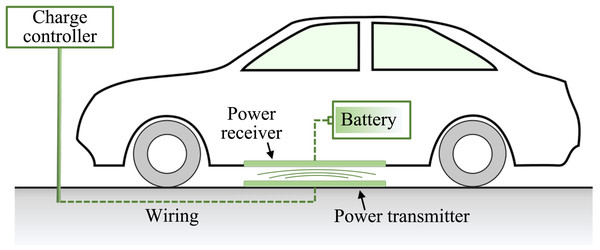Figure 1: Wireless power transfer (WPT) for electric vehicles (EVs). When the power transmitter and receiver coils are kept close to each other, electric energy can be used to charge the battery. The alignment of the two coils is significant for the high performance and efficiency of the WPT.   Download full-size image DOI: 10.7717/peerjcs.567/fig-1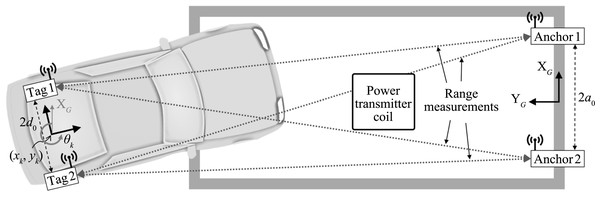Figure 2: Dual-anchor and dual-tag (DADT) ultrawideband (UWB)-based localization system. Two UWB anchors are placed on both corners of the parking slot and two UWB tags are mounted on the vehicle. The observability of the vehicle’s pose estimated by the proposed DADT UWB system is analyzed based on the Fisher information matrix.   Download full-size image DOI: 10.7717/peerjcs.567/fig-2

Localization technologies that can be applied in parking lot environments have been developed based on mono cameras (Hu et al., 2019; Panev et al., 2019; Yu et al., 2020), depth cameras (Zhao et al., 2020), lidar (Tao et al., 2018), and radio frequency (RF) fingerprinting of WiFi signals (Gao, He & Li, 2018), but they still have some limitations. Most camera-based localization technologies are based on parking line recognition. However, parking lines are usually not standardized and may not even be drawn. These methods are also sensitive to lighting changes at night and do not operate well in dark indoor parking lots. Lidar is useful for finding vacant parking spaces, but it is difficult to estimate the relative position from the transmitting coil because it is difficult for lidar to recognize the position of the transmitting coil.

The use of UWB sensors can overcome the limitations of cameras and lidar sensors. The proposed DADT localization method only needs to know the positions of two anchors and the transmitting coil installed in the parking slot. Thus, it does not require an inconvenient process of building a high-precision map with cameras or lidar sensors. As the UWB sensor is based on RF signals, it is unaffected by changes in lighting and robust against dynamic obstacles such as vehicles and pedestrians. In addition, if a pair of UWB sensors has a clear line of sight, the distance between them can be precisely measured with an error of approximately 0.05 m- 0.1 m. Due to the economics of UWB sensors, many automotive makers have plans to use UWB sensors in vehicles soon. Therefore, it is possible to implement a precise localization applicable to WPT for EVs with economical cost.

## Proposed Ultrawideband (UWB)-based localization

### Dual-Anchor and dual-tag (DADT) UWB localization and observability analysis

This section describes a novel dual-anchor and dual-tag (DADT) UWB-based localization method that can precisely estimate the pose of a vehicle near a parking area. The key idea of the proposed DADT UWB-based localization is shown in Fig. 2. Two anchors are installed on the charging station, and two tags are mounted on the vehicle. The two tags should be placed so that the pose of the vehicle is always observable with only the distance measurements of the DADT UWB system. The location of the UWB anchors should be known in advance. Thus, the two anchors are placed on both corners of the parking slot so that the location of the anchor can be easily identified.

To show the effectiveness of the proposed DADT UWB sensor system, the condition in which the vehicle’s pose can be uniquely determined by the DADT UWB sensor system is analytically derived. Then, observability analysis based on FIM is performed on the proposed DADT UWB sensor system.

#### Existence and Uniqueness Solution to DADT UWB localization

We denote the vehicle pose state vector at a time step k by xk = [xkykθk]T, the two UWB anchor position vectors by ${\mathbf{a}}_{1}={\left[{a}_{1x},{a}_{1y}\right]}^{T}$ and ${\mathbf{a}}_{2}={\left[{a}_{2x},{a}_{2y}\right]}^{T}$, and the two UWB tag position vectors by ${\mathbf{t}}_{1}={\left[{t}_{1x},{t}_{1y}\right]}^{T}$ and ${\mathbf{t}}_{2}={\left[{t}_{2x},{t}_{2y}\right]}^{T}$. For simplicity, let us assume that the two anchors are placed at ${\mathbf{a}}_{1}={\left[{a}_{0},0\right]}^{T}$ and ${\mathbf{a}}_{2}={\left[-{a}_{0},0\right]}^{T}$, as shown in Fig. 2. The position vectors of the two tags can then be represented in the global frame $\left({\text{X}}_{G},{\text{Y}}_{G}\right)$ as ${\mathbf{t}}_{1}=\left[\begin{array}{c}\hfill {t}_{1x}\hfill \\ \hfill {t}_{1y}\hfill \end{array}\right]=\left[\begin{array}{c}\hfill {x}_{k}-{d}_{0}sin{\theta }_{k}\hfill \\ \hfill {y}_{k}+{d}_{0}cos{\theta }_{k}\hfill \end{array}\right],$ ${\mathbf{t}}_{2}=\left[\begin{array}{c}\hfill {t}_{2x}\hfill \\ \hfill {t}_{2y}\hfill \end{array}\right]=\left[\begin{array}{c}\hfill {x}_{k}+{d}_{0}sin{\theta }_{k}\hfill \\ \hfill {y}_{k}-{d}_{0}cos{\theta }_{k}\hfill \end{array}\right].$ The measurement model of the DADT UWB system can then be derived as $h\left({\mathbf{x}}_{t},{a}_{0},{d}_{0}\right)=\left[\begin{array}{c}\hfill \sqrt{{\left({x}_{k}-{d}_{0}sin{\theta }_{k}-{a}_{0}\right)}^{2}+{\left({y}_{k}+{d}_{0}cos{\theta }_{k}\right)}^{2}}\hfill \\ \hfill \sqrt{{\left({x}_{k}+{d}_{0}sin{\theta }_{k}-{a}_{0}\right)}^{2}+{\left({y}_{k}-{d}_{0}cos{\theta }_{k}\right)}^{2}}\hfill \\ \hfill \sqrt{{\left({x}_{k}-{d}_{0}sin{\theta }_{k}+{a}_{0}\right)}^{2}+{\left({y}_{k}+{d}_{0}cos{\theta }_{k}\right)}^{2}}\hfill \\ \hfill \sqrt{{\left({x}_{k}+{d}_{0}sin{\theta }_{k}+{a}_{0}\right)}^{2}+{\left({y}_{k}-{d}_{0}cos{\theta }_{k}\right)}^{2}}\hfill \\ \hfill \hfill \end{array}\right],$ and Eq. (3) can be represented as ${z}_{11}^{2}={\left({x}_{k}-{d}_{0}sin{\theta }_{k}-{a}_{0}\right)}^{2}+{\left({y}_{k}+{d}_{0}cos{\theta }_{k}\right)}^{2}$ ${z}_{12}^{2}={\left({x}_{k}+{d}_{0}sin{\theta }_{k}-{a}_{0}\right)}^{2}+{\left({y}_{k}-{d}_{0}cos{\theta }_{k}\right)}^{2}$ ${z}_{21}^{2}={\left({x}_{k}-{d}_{0}sin{\theta }_{k}+{a}_{0}\right)}^{2}+{\left({y}_{k}+{d}_{0}cos{\theta }_{k}\right)}^{2}$ ${z}_{22}^{2}={\left({x}_{k}+{d}_{0}sin{\theta }_{k}+{a}_{0}\right)}^{2}+{\left({y}_{k}-{d}_{0}cos{\theta }_{k}\right)}^{2},$ where zij is the distance measurement value by the ith anchor and jth tag. The vehicle pose as determined by the DADT UWB system can be found by solving Eqs. (4a)(4d) for ${\mathbf{x}}_{k}={\left[{x}_{k},{y}_{k},{\theta }_{k}\right]}^{T}$. From Eqs. (4a) and (4c), xk and yk can be uniquely determined by ${x}_{k}={d}_{0}sin{\theta }_{k}+\frac{1}{4{a}_{0}}\left({z}_{21}^{2}-{z}_{11}^{2}\right)$ ${y}_{k}=-{d}_{0}cos{\theta }_{k}+\sqrt{{z}_{11}^{2}-{\left[\frac{1}{4{a}_{0}}\left({z}_{21}^{2}-{z}_{11}^{2}\right)-{a}_{0}\right]}^{2}},$ under the condition that ${y}_{k}+{d}_{0}cos{\theta }_{k}>0.$ In a similar manner, using Eqs. (4b) and (4d), xk and yk have another equivalent form as follows: ${x}_{k}=-{d}_{0}sin{\theta }_{k}+\frac{1}{4{a}_{0}}\left({z}_{22}^{2}-{z}_{12}^{2}\right)$ ${y}_{k}={d}_{0}cos{\theta }_{k}+\sqrt{{z}_{12}^{2}-{\left[\frac{1}{4{a}_{0}}\left({z}_{22}^{2}-{z}_{12}^{2}\right)-{a}_{0}\right]}^{2}},$ under the condition that ${y}_{k}-{d}_{0}cos{\theta }_{k}>0.$ The two conditions Eqs. (6) and (8) can be satisfied the two tags are on the YG > 0 region. Subtracting Eqs. (7a) from (5a) and rearranging with respect to sinθk gives $sin{\theta }_{k}=\frac{1}{8{a}_{0}{d}_{0}}\left({z}_{11}^{2}-{z}_{12}^{2}-{z}_{21}^{2}+{z}_{22}^{2}\right).$ From Eqs. (5b) and (7b), cosθk can be expressed by $cos{\theta }_{k}=\frac{1}{2{d}_{0}}\left(\sqrt{{z}_{11}^{2}-{\left[\frac{1}{4{a}_{0}}\left({z}_{21}^{2}-{z}_{11}^{2}\right)-{a}_{0}\right]}^{2}}-\sqrt{{z}_{12}^{2}-{\left[\frac{1}{4{a}_{0}}\left({z}_{22}^{2}-{z}_{12}^{2}\right)-{a}_{0}\right]}^{2}}\right).$ Using Eqs. (9) and (10), θk can be found as ${\theta }_{k}=arctan\left(\frac{1}{4{a}_{0}}\frac{{z}_{11}^{2}-{z}_{12}^{2}-{z}_{21}^{2}+{z}_{22}^{2}}{\sqrt{{z}_{11}^{2}-{\left[\frac{1}{4{a}_{0}}\left({z}_{21}^{2}-{z}_{11}^{2}\right)-{a}_{0}\right]}^{2}}-\sqrt{{z}_{12}^{2}-{\left[\frac{1}{4{a}_{0}}\left({z}_{22}^{2}-{z}_{12}^{2}\right)-{a}_{0}\right]}^{2}}}\right).$ Therefore, the vehicle pose ${\mathbf{x}}_{k}={\left[{x}_{k},{y}_{k},{\theta }_{k}\right]}^{T}$ can be uniquely determined by Eqs. (7a), (7b) and Eq. (11) under the condition that yk > d0.

#### Observation analysis based on the Fisher information matrix (FIM)

The uncertainty of the pose of the vehicle estimated by UWB distance measurements is determined by the geometric distribution of the anchors fixed on the parking lot and the tags mounted on the vehicle. To estimate the amount of uncertainty about the vehicle pose estimated by the proposed DADT UWB system, FIM-based observability analysis is performed as follows. The FIM can be defined as (Lee et al., 2015) $\mathcal{F}\triangleq {\mathbf{H}}^{T}{\mathbf{W}}^{-1}\mathbf{H}$ where H is the Jacobian of $h\left({\mathbf{x}}_{t},{a}_{0},{d}_{0}\right)$ in Eq. (3) with respect to the vehicle state xk and W is a covariance matrix of the UWB measurement noise. The sufficient and necessary condition for the DADT UWB localization system to be fully observable is that the FIM defined in Eq. (12) should be positive definite. The positive definiteness of the FIM is equivalent to the full column rank of the Jacobian H. Therefore, it can be found that the DADT UWB localization system is fully observable from the UWB distance measurements if and only if H has a full column rank. The Jacobian H can be computed as $\mathbf{H}=\frac{\partial h\left({\mathbf{x}}_{k},{a}_{0},{d}_{0}\right)}{\partial {\mathbf{x}}_{k}}=\left[\begin{array}{cc}\hfill \frac{1}{{m}_{11}}\left({x}_{k}-{a}_{0}-{d}_{0}sin{\theta }_{k}\right)\hfill & \hfill \frac{1}{{m}_{12}}\left({x}_{k}-{a}_{0}+{d}_{0}sin{\theta }_{k}\right)\hfill \\ \hfill \frac{1}{{m}_{11}}\left({y}_{k}+{d}_{0}cos{\theta }_{k}\right)\hfill & \hfill \frac{1}{{m}_{12}}\left({y}_{k}-{d}_{0}cos{\theta }_{k}\right)\hfill \\ \hfill -\frac{{d}_{0}}{{m}_{11}}\left(\left({x}_{k}-{a}_{0}\right)cos{\theta }_{k}+{y}_{k}sin\theta \right)\hfill & \hfill \frac{{d}_{0}}{{m}_{12}}\left(\left({x}_{k}-{a}_{0}\right)cos{\theta }_{k}+{y}_{k}sin\theta \right)\hfill \end{array}\right\begin{array}{cc}\hfill \frac{1}{{m}_{21}}\left({x}_{k}+{a}_{0}-{d}_{0}sin{\theta }_{k}\right)\hfill & \hfill \frac{1}{{m}_{22}}\left({x}_{k}+{a}_{0}+{d}_{0}sin{\theta }_{k}\right)\hfill \\ \hfill \frac{1}{{m}_{21}}\left({y}_{k}+{d}_{0}cos{\theta }_{k}\right)\hfill & \hfill \frac{1}{{m}_{22}}\left({y}_{k}-{d}_{0}cos{\theta }_{k}\right)\hfill \\ \hfill -\frac{{d}_{0}}{{m}_{21}}\left(\left({x}_{k}+{a}_{0}\right)cos{\theta }_{k}+{y}_{k}sin\theta \right)\hfill & \hfill \frac{{d}_{0}}{{m}_{22}}\left(\left({x}_{k}+{a}_{0}\right)cos{\theta }_{k}+{y}_{k}sin\theta \right)\hfill \\ \hfill \hfill \end{array}].$ The Jacobian presented in Eq. (13) can also be represented in a reduced row echelon form using the Gaussian elimination process as follows: $\left[\begin{array}{cccc}\hfill 1\hfill & \hfill 0\hfill & \hfill 0\hfill & \hfill \frac{-{m}_{11}\left({y}_{k}-dcos{\theta }_{k}\right)\left(\left({x}_{k}+a\right)cos{\theta }_{k}+{y}_{k}sin{\theta }_{k}\right)}{{m}_{22}\left({y}_{k}+dcos{\theta }_{k}\right)\left(\left({x}_{k}-a\right)cos{\theta }_{k}+ysin{\theta }_{k}\right)}\hfill \\ \hfill 0\hfill & \hfill 1\hfill & \hfill 0\hfill & \hfill \frac{{m}_{12}\left(\left({x}_{k}+a\right)cos{\theta }_{k}+ysin{\theta }_{k}\right)}{{m}_{22}\left(\left({x}_{k}-a\right)cos{\theta }_{k}+ysin{\theta }_{k}\right)}\hfill \\ \hfill 0\hfill & \hfill 0\hfill & \hfill 1\hfill & \hfill \frac{{m}_{21}\left({y}_{k}-dcos{\theta }_{k}\right)}{{m}_{22}\left({y}_{k}+dcos{\theta }_{k}\right)}\hfill \\ \hfill \hfill \end{array}\right].$ As seen in Eq. (14), the column rank is 3, which is the full rank.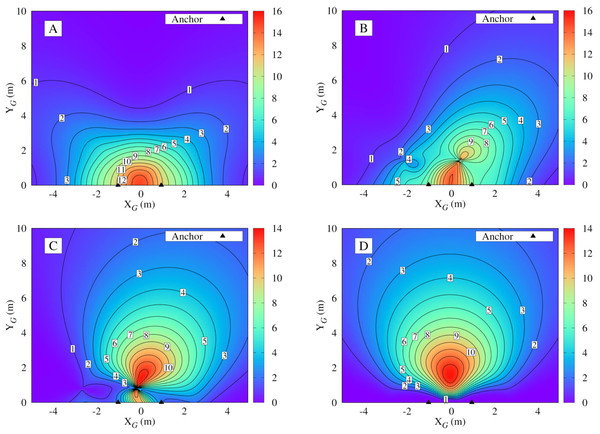Figure 3: Numerical distribution of the determinant of the Fisher information matrix in Eq. (12) around the UWB anchors placed at a 1 = 1 , 0 T and a 2 = − 1 , 0 T with different vehicle’s heading angles for every position. (A) θk = 0 deg or 180 deg; (B) θk = 30 deg or 210 deg; (C) θk = 60 deg or 240 deg; (D) θk = 90 deg or 270 deg.   Download full-size image DOI: 10.7717/peerjcs.567/fig-3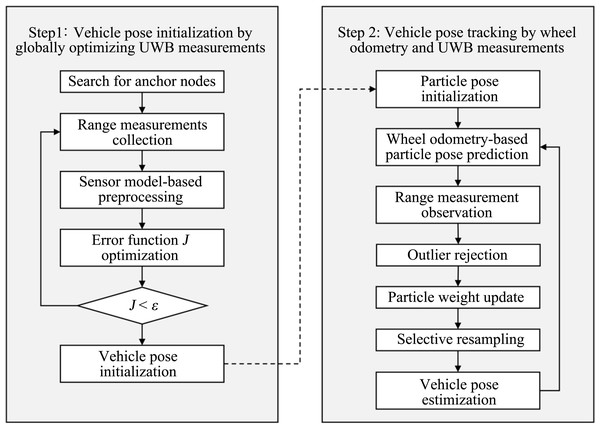Figure 4: Flowchart of the proposed DADT UWB localization algorithm. In the first step, the vehicle’s pose is initialized by globally optimizing the UWB distance measurements. In the second step, based on the initialized vehicle pose, the wheel odometry and UWB distance measurement collected as the vehicle moves are fused to estimate the pose of the vehicle in real time.   Download full-size image DOI: 10.7717/peerjcs.567/fig-4

The determinant value of the FIM represents the amount of Fisher information that can be observed for vehicle pose state variables; i.e., as the determinant of the FIM increases, the vehicle pose can be estimated with higher accuracy. Figure 3 shows the numerical distribution of the determinant of the FIM around the UWB anchors placed at ${\mathbf{a}}_{1}={\left[1,0\right]}^{T}$ and ${\mathbf{a}}_{2}={\left[-1,0\right]}^{T}$, when the vehicle heading is θk = 0 deg, 30 deg, 60 deg, and 90 deg for every position. In Fig. 3, the covariance matrix W in Eq. (12) is assumed to be an identity matrix in order to compare the determinant value depending only on the positions. As shown in Fig. 2, when the vehicle approaches the parking slot, the heading angle θk is 90 deg for back-in parking and 270 deg for front-end parking. By comparing Fig. 3, it can be seen that when the heading angle is 90 deg or 270 deg, the determinant of the FIM has the largest distribution. This means that for anchors placed at both corners of the parking lot slot, installing the two tags in a direction perpendicular to the vehicle’s moving direction maximizes the amount of Fisher information under the assumption that back-in parking (θk = 90deg) or front-end parking (θk = 270deg) is performed.

### Vehicle pose estimation based on the DADT UWB system

The proposed DADT UWB localization algorithm consists of two major steps, as shown in Fig. 4. In the first step, when the EV approaches the parking area where the UWB tag and anchor can communicate, distance measurements between UWB anchors and tags are collected. Subsequently, the pose of the EV is initialized through global optimization of the UWB measurements. In the second step, based on the initialized vehicle pose, wheel odometry and UWB distance data collected as the vehicle moves are fused to estimate the pose of the vehicle in real time. The details are given in the following subsections.

#### Vehicle pose initialization by globally optimizing UWB measurements

The purpose of this step is to quickly find the approximate initial pose of the EV using only UWB distance measurements under the assumption that no prior information about the EV’s pose is available. From the mathematical analysis of the proposed DADT UWB system, the EV pose can always be uniquely determined by the DADT UWB system in the area of y > d0. Therefore, it is possible to predict the sensor measurement value from the measurement model as formulated in Eq. (3) and determine the initial pose of the vehicle through global optimization between the predicted value and the actual measurement value.

We propose a particle swarm optimization (PSO) (Kennedy, Eberhart & Shi, 2001)-based global optimization algorithm that can quickly search for suboptimal solutions to find the initial pose. Each particle in PSO is considered a potential solution, i.e., a vehicle pose state vector, and searches for a given solution space. The position of each particle is iteratively updated based on the experience of the particle and its neighbors and converges toward the optimal solution quickly.

The PSO-based vehicle pose initialization method is as follows. When a vehicle approaches a parking area where communication between UWB anchors and tags is possible, UWB distance measurements between each UWB tag and anchor pair are sampled. When a certain number of measurements is collected, the average value is estimated by removing outliers. The error function J for global optimization is defined as follows. $J={\left[h\left({\mathbf{x}}_{t},{a}_{0},{d}_{0}\right)-{\mathbf{z}}_{k}\right]}^{T}\left[h\left({\mathbf{x}}_{t},{a}_{0},{d}_{0}\right)-{\mathbf{z}}_{k}\right],$ where zk is a measurement vector. For the region where y > d0, the PSO finds the initial vehicle pose ${\mathbf{x}}_{0}={\left[{x}_{0},{y}_{0},{\theta }_{0}\right]}^{T}$ whose error function J value is less than or equal to a specific threshold ϵ. The threshold is determined by considering the variance of UWB measurements.

#### Vehicle pose tracking by fusing odometry and UWB measurements

To estimate the vehicle’s pose precisely as the vehicle moves, the UWB distance measurements and wheel odometry data are fused through a particle filter. The method of estimating the vehicle’s pose through the particle filter is shown in the right block of Fig. 4, and the details of each part are as follows.

In particle filter-based localization, a group of particles represents the probability distribution of vehicle states, with each particle ${\mathbf{x}}_{k}^{\left[m\right]}$ representing a possible state, where [m] indicates a particle index. When the initial pose state of the EV is determined by the PSO, the particles are initialized to have a Gaussian distribution. The mean of the distribution is set to the initial pose state determined by the PSO.

The motion model of the vehicle is ${\mathbf{x}}_{k}=f\left({\mathbf{x}}_{k-1},{\mathbf{u}}_{k}\right)+{\mathbf{e}}_{u},$ where uk is a control input vector and eu is normally distributed process noise with zero mean. Given the current vehicle pose xk and the positions of the anchors fixed at a1 = [a0, 0]T and a2 = [ − a0, 0]T, the observation model of the DADT UWB system can be written as ${\mathbf{z}}_{k}=h\left({\mathbf{x}}_{k},{a}_{0},{d}_{0}\right)+{\mathbf{e}}_{v}$ where ev is normally distributed Gaussian noise with zero mean.

The vehicle pose ${\mathbf{x}}_{k}^{\left[m\right]}$ is predicted by taking the wheel odometry into consideration, which is denoted by ${\mathbf{x}}_{k}^{\left[m\right]}\sim p\left({\mathbf{x}}_{k}\mid {\mathbf{x}}_{1:k-1}^{\left[m\right]},{\mathbf{u}}_{k}\right)$. The importance weight is computed by (Grisetti, Stachniss & Burgard, 2007) ${\omega }_{k}^{\left[m\right]}=\frac{1}{\sqrt{2\pi \mathbf{Q}}}exp\left[-\frac{1}{2}{\left({\mathbf{z}}_{k}-{\stackrel{ˆ}{\mathbf{z}}}_{k}^{\left[m\right]}\right)}^{T}{\left({\mathbf{Q}}_{k}\right)}^{-1}\left({\mathbf{z}}_{k}-{\stackrel{ˆ}{\mathbf{z}}}_{k}^{\left[m\right]}\right)\right],$ where ${\stackrel{ˆ}{\mathbf{z}}}_{k}^{\left[m\right]}$ is a predicted measurement and Qk is the covariance of the anchors’ positions. In the process of particle filtering, the importance weight of some particles can gradually become low, and particles with lower importance weights have little effect in estimating the vehicle pose states. To prevent this effect, the particles are resampled in proportion to the weight of each particle. The number of effective particles given by ${N}_{eff}=\frac{1}{\sum _{m=1}^{M}{\left({\omega }_{k}^{\left[m\right]}\right)}^{2}},$ where M is the total number of particles. When the number of effective particle is less than 50% of the total number of particles, the weights of all particles are uniformly reset after resampling particles.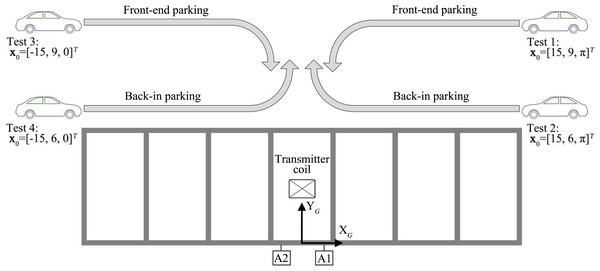Figure 5: Four simulation tests. The vehicle starts from four different positions and moves along the path for front-end parking or back-in parking.   Download full-size image DOI: 10.7717/peerjcs.567/fig-5

## Simulation and experimental results

### Simulation results

To verify the performance of the proposed DADT UWB localization method, we perform the following simulations: (1) Initialize the vehicle’s pose by globally optimizing the error function defined in Eq. (15); (2) estimate the vehicle’s pose by fusing wheel odometry and UWB measurements. Figure 5 shows the simulation scenario. The vehicle starts from four different positions and moves along the path for front-end parking or back-in parking. Considering the actual dimensions of the vehicle and the parking space environment, the distance between the UWB tags mounted on the vehicle is set to 1.36 m, i.e., d0 = 0.68 and the two UWB anchors are placed at a1 = [1, 0]T and a2 = [ − 1, 0]T. The wheel odometry noise and UWB measurement noises are assumed to be sampled from the normal distributions denoted by ${\mathbf{e}}_{u}\sim N\left(0,{\sigma }_{u}^{2}\right)$ and ${\mathbf{v}}_{u}\sim N\left(0,{\sigma }_{v}^{2}\right)$ where σu = 0.35 m/s and σv = 0.10 m.

Table 1 shows the initial pose estimation results by the proposed global optimizing UWB measurements for the four selected poses. To show the effectiveness of the proposed vehicle’s pose initialization method, the results of the Levenberg–Marquardt method (Moré, 1978), which is a widely used method of optimization of the least square problem, are compared with the results of the proposed DADT method. The pose error $\mathcal{E}$ between the estimated initial pose x0 and the ground truth pose xGT defined by $\mathcal{E}=\parallel {\mathbf{x}}_{0}-{\mathbf{x}}_{GT}\parallel .$ Since the Levenberg–Marquardt method is a local optimization method, it has a limitation in that it cannot find the initial position when it converges to a local minimum. As seen in Table 1, the Levenberg–Marquardt method fails to find the vehicle’s initial pose. However, the proposed initial pose estimation method can precisely find the initial pose for all the tests. These results are consistent with the mathematical analysis of the DADT UWB localization system.

Figures 69 show the results of estimating the vehicle’s pose continuously from the initial pose through the fusion of the vehicle’s odometry data and the UWB distance measurements under the particle filter framework with a fixed number of particles, M = 100. As seen from the results, the error of the odometry increases as the vehicle moves, whereas the trajectories estimated by the proposed DADT method match the ground truth trajectories in all four cases. Figure 10 shows boxplots for each test, including the mean, minimum, maximum, and standard deviation of the errors. Table 2 shows the numerical values corresponding to the boxplots. The results show that the proposed DADT method keeps the mean error of the vehicle’s position under 0.1 m.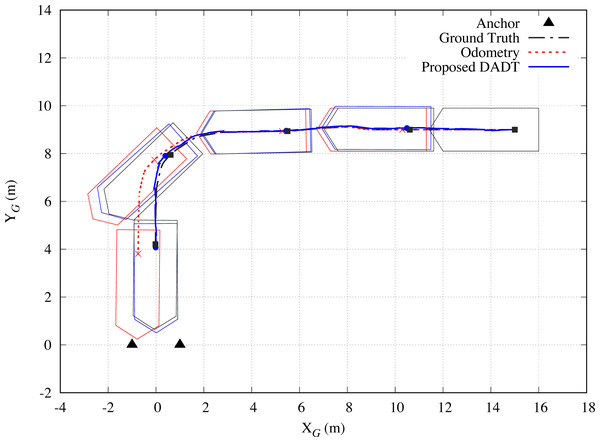Figure 6: Simulation results of Test 1. The vehicle starts from its initial pose x 0 = 15 , 9 , π T and moves along the path for front-end parking.   Download full-size image DOI: 10.7717/peerjcs.567/fig-6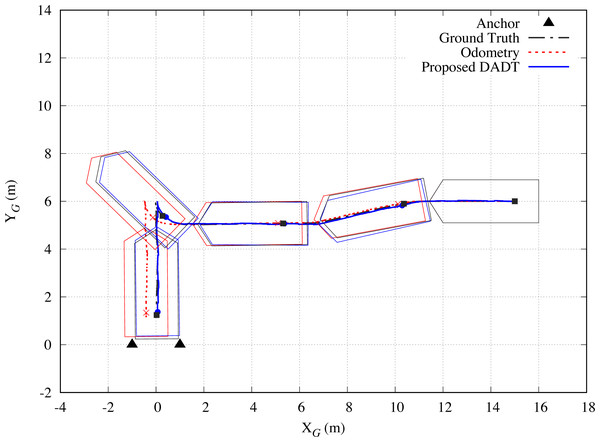Figure 7: Simulation results of Test 2. The vehicle starts from its initial pose x 0 = 15 , 6 , π T and moves along the path for back-in parking.   Download full-size image DOI: 10.7717/peerjcs.567/fig-7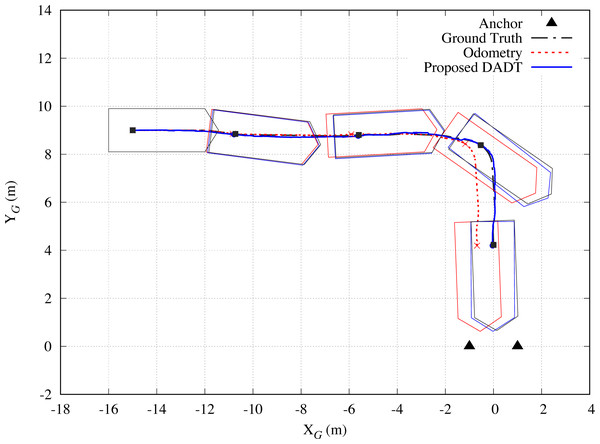Figure 8: Simulation results of Test 3. The vehicle starts from its initial pose x 0 = − 15 , 9 , 0 T and moves along the path for front-end parking.   Download full-size image DOI: 10.7717/peerjcs.567/fig-8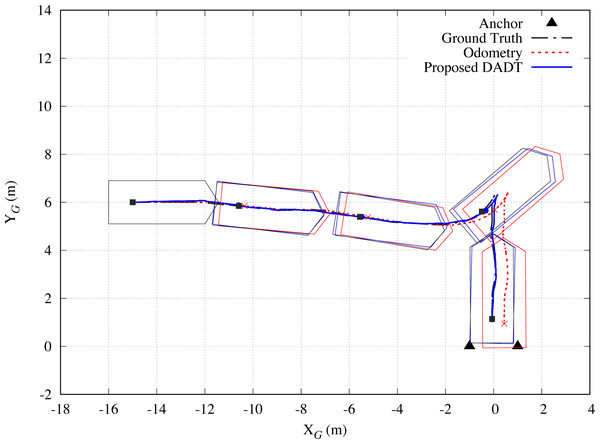Figure 9: Simulation results of Test 4. The vehicle starts from its initial pose x 0 = − 15 , 6 , 0 T and moves along the path for back-in parking.   Download full-size image DOI: 10.7717/peerjcs.567/fig-9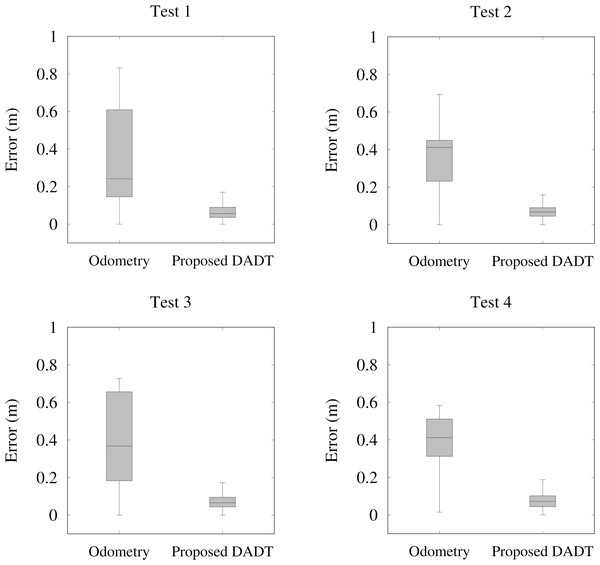Figure 10: Boxplots of the simulation results.   Download full-size image DOI: 10.7717/peerjcs.567/fig-10

### Experimental results

The proposed DADT UWB-based localization method is tested with a real vehicle. The tests are performed with the UWB modules manufactured by Pozyx (Pozyx, 2021), which have a maximum measurable distance of 30 m and an update rate of 60 Hz. As shown in Fig. 11, two UWB tags are mounted on the vehicle roof, and two UWB anchors are installed near both corners of the parking slot. The UWB anchors are installed at a height of 1.8 m to maintain line-of-sight communication with the UWB tags mounted on the vehicle. The positions where the UWB anchors and tags are installed are set to be the same as in the simulation. To evaluate the error of the proposed method, a differential global positioning system (DGPS) receiver module with centimeter-level accuracy is also mounted on the vehicle. The proposed localization algorithm is implemented to perform real-time computation at 10 Hz on an NVIDIA Nano Jetson board (NVIDIA, 2021). Figure 12 shows snapshots of the experiment with a real vehicle performing front-end parking.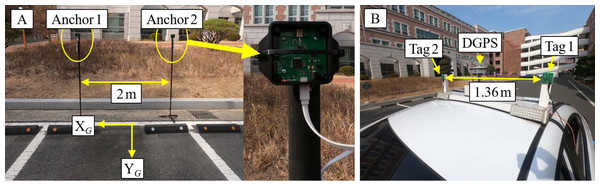Figure 11: Experimental setup. (A) Two UWB anchors are installed near both corners of the parkingslot. (B) two UWB tags and DGPS are mounted on the vehicle roof.   Download full-size image DOI: 10.7717/peerjcs.567/fig-11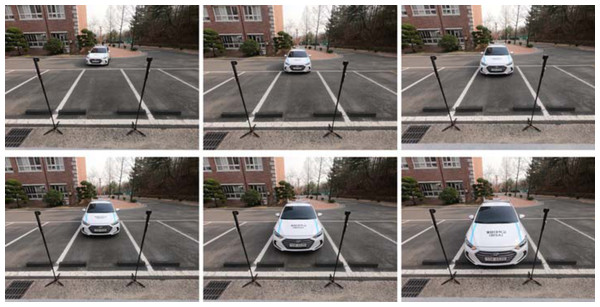Figure 12: Snapshots of the experiment with a real vehicle. The vehicle is performing front-end parking.   Download full-size image DOI: 10.7717/peerjcs.567/fig-12

Figures 1314 show the experimental results with the actual vehicle. As shown in the experimental results, the error generated by the wheel odometry increases due to the slip of the wheels when moving along a curved path. However, the proposed DADT UWB-based method precisely corrects the vehicle’s pose using the UWB distance measurements. Table 3 shows that the errors are within 0.1 m at the final parked position. The proposed DADT UWB-based localization method can be sufficiently applied to WPT.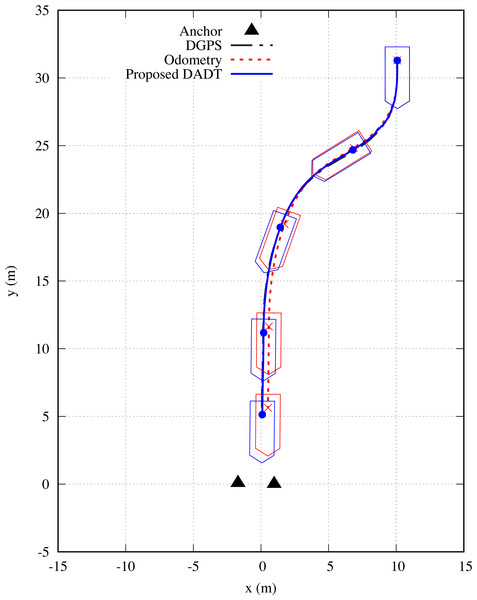Figure 13: Results of Exp. 1. The black dashed-dotted line shows the DGPS trajectory, the red dotted line shows the odometry trajectory, and the blue solid line shows the proposed DADT UWB-based localization results.   Download full-size image DOI: 10.7717/peerjcs.567/fig-13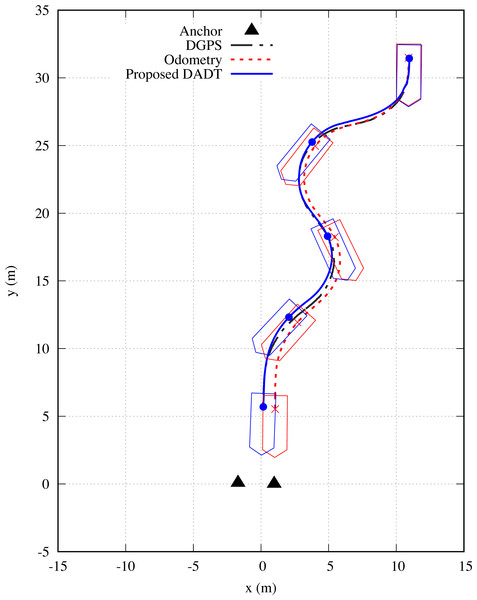Figure 14: Results of Exp. 2. The black dashed-dotted line shows the DGPS trajectory, the red dotted line shows the odometry trajectory, and the blue solid line shows the proposed DADT UWB-based localization results.   Download full-size image DOI: 10.7717/peerjcs.567/fig-14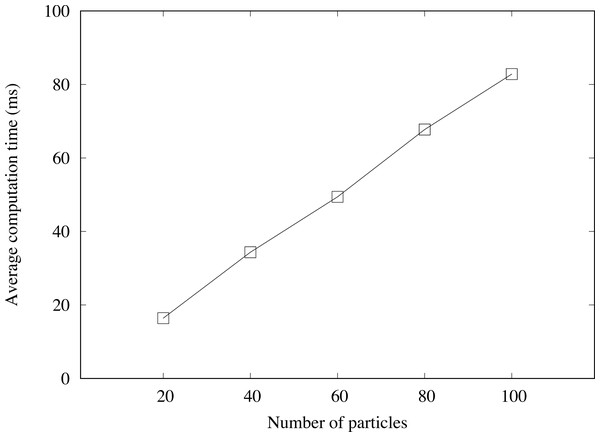Figure 15: Average computation time required to update the vehicle’s pose at each time instant.   Download full-size image DOI: 10.7717/peerjcs.567/fig-15

Through the experiments, the average computation time required to update the vehicle’s pose at each time instant is estimated while increasing the number of particles from 20 to 100. Figure 15 shows the average computation time estimated by an NVIDIA Nano Jetson board. The computation time grows linearly as the number of particles increases. However, even when 100 particles are used, it can be operated in real time at a rate of 10 Hz.

## Conclusions

This paper proposed a novel short-range precise localization method using a DADT UWB sensor system for application to a WPT system. An observability analysis of the proposed DADT UWB sensor system consisting of two anchors and two tags was performed based on the FIM. The proposed localization algorithm determines the vehicle’s initial pose by globally optimizing the collected UWB distance measurements and estimates the vehicle’s pose by fusing the vehicle’s wheel odometry data and the UWB distance measurements. The effectiveness of the proposed method was confirmed through various simulations and real vehicle-based experiments.

## Supplemental Information

### Source code (C++) for the proposed DADT UWB localization algorithm

The source code includes (i) DADT UWB-based localization algorithm, (ii) particle filter framework to estimate a vehicle’s pose.

### Competing Interests

The authors declare there are no competing interests.

### Author Contributions

Seung-Mok Lee conceived and designed the experiments, performed the experiments, analyzed the data, performed the computation work, prepared figures and/or tables, authored or reviewed drafts of the paper, and approved the final draft.

### Data Availability

The following information was supplied regarding data availability:

The source code is available in the Supplementary File.

### Funding

This work was supported by the National Research Foundation of Korea (NRF) grant funded by the Korea government (MSIT) (No. NRF-2018R1C1B5038763). The funders had no role in study design, data collection and analysis, decision to publish, or preparation of the manuscript.